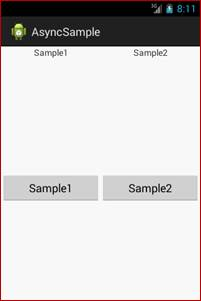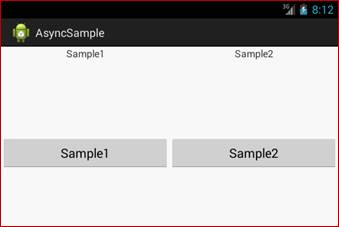#### How to set layout height and weight in percentage android?

Total Post:47

Points:331
3138  View(s)
Ratings:
Rate this:
How to set layout  height and weight in percentage android
1.Post:48

Points:336
##### Re: How to set layout height and weight in percentage android?

You need to add weight in your controls xml file. Here I am giving a sample, which help you to set height and weight in percentage.

`   <LinearLayout xmlns:android="http://schemas.android.com/apk/res/android"     xmlns:tools="http://schemas.android.com/tools"     android:layout_width="match_parent"     android:layout_height="match_parent"     android:orientation="vertical"   tools:context=".MainActivity" >   <!--weight=".5" means 50 percantage -->   <LinearLayout       android:layout_width="fill_parent"       android:layout_height="0dp"       android:layout_weight=".5"       android:orientation="horizontal" >       <TextView           android:layout_width="0dp"           android:layout_height="wrap_content"           android:layout_weight=".5"           android:gravity="center"           android:text="Sample1" />        <TextView            android:layout_width="0dp"            android:layout_height="wrap_content"            android:layout_weight=".5"            android:gravity="center"            android:text="Sample2" />    </LinearLayout>    <LinearLayout        android:layout_width="fill_parent"        android:layout_height="0dp"        android:layout_weight=".5"        android:orientation="horizontal" >        <Button            android:layout_width="0dp"            android:layout_height="wrap_content"            android:layout_weight=".5"            android:gravity="center"            android:text="Sample1" />        <Button            android:layout_width="0dp"            android:layout_height="wrap_content"            android:layout_weight=".5"            android:gravity="center"            android:text="Sample2" />    </LinearLayout></LinearLayout>`Modified On Apr-09-2018 01:40:09 AM